Next: 12.4 The Exponential Function Up: 12. Power Series Previous: 12.2 Radius of Convergence   Index

# 12.3 Differentiation of Power Series

If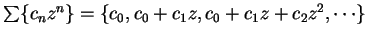is a power series, then the series obtained by differentiating the terms of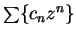isThis is not a power series, but its translate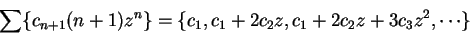is.

12.15   Definition (Formal derivative.) Ifis a power series, then the formal derivative ofis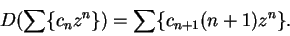I will sometimes write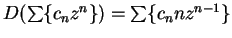when I think this will cause no confusion.

12.16   Examples.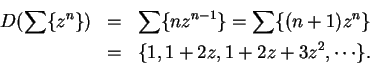orOur fundamental theorem on power series is:

12.17   Theorem (Differentiation theorem.) Let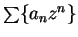be a power series. Then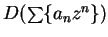andhave the same radius of convergence. The function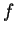associated withis differentiable in the disc of convergence, and the function represented byagrees with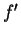on the disc of convergence.

The proof is rather technical, and I will postpone it until section 12.8. I will derive some consequences of it before proving it (to convince you that it is worth proving).

12.18   Example. We know that the geometric serieshas radius of convergence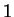and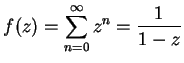for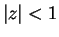. The differentiation theorem says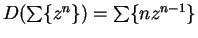also has radius of convergence, and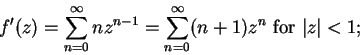i.e.,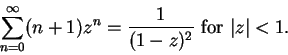We can apply the theorem again and get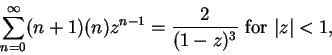or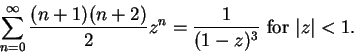Another differentiation gives us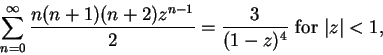or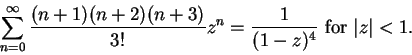The pattern is clear, and I omit the induction proof that for all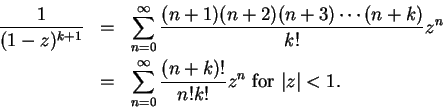12.19   Exercise. By assuming the differentiation theorem, we've shown that the series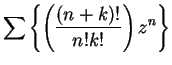has radius of convergencefor all. Verify this directly.

12.20   Exercise. A Find formulas for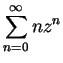and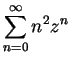that are valid for. (You may assume the differentiation theorem.)

12.21   Example. By the differentiation theorem, if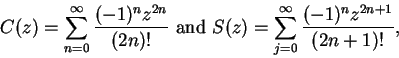then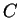andare differentiable on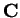and, and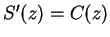. (We saw in earlier examples that both series have radius of convergence, and that the formal derivatives satisfy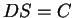and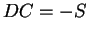.) Also, clearly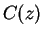and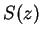are real when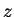is real. The discussion in example 10.3 then shows that for real,andagree with the cosine and sine functions you discussed in your previous calculus course, and in particular that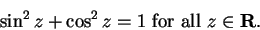Next: 12.4 The Exponential Function Up: 12. Power Series Previous: 12.2 Radius of Convergence   Index